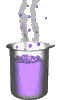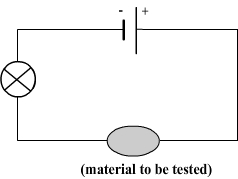Science class online
Chemistry and physics lessons

# Electricity

Free online electricity lessons for elementary school,  middle school and high school.Science class Chemistry Electricity Optics Mechanics Electricity lessons Electrical components -Two-terminal electronic components - Basic electrical components - Diodes - What's a resistor ? - How to determine a resistor value ? - Resistors effects in circuits - How to use a resistor ? - Characteristic curve of a resistor How to build simple circuits and draw diagrams - How to build a basic electrical circuit - How to draw diagrams of electric circuits The electric current - Conductors and insulators - Direction of electric current in a circuit - The dangers of electricity - Current intensity - units how to measure current ? The Voltage - The voltage and its units - How to measure a voltage ? - Voltage in open and closed circuits - Rated current and voltage for a lamp Alternating voltage and current - Alternating currents effects on led - What are alternating current and voltage ? - Periodic alternating voltage and its properties - Oscilloscope - Oscillogram - Frequency - Measuring RMS voltage with a voltmeter Serie circuits - What's a serie circuit ? - Series circuit properties - Short circuit in  series circuit - Current law in series - circuits - Voltage law in series circuits Parallel circuits - What's a parallel circuit ? - Some parallel circuits properties - Short circuits in parallel circuits - Nodes and branches in parallel circuits - Current laws in parallel circuits - Voltage laws in parallel circuits Laws of electricity - Ohm's law - Current laws in series circuits - Voltage laws in series circuits - Current laws in parallel circuits - Voltage laws in parallel circuits Generating electricity - Voltage for coil wires What is an alternator ? - Alternating currents and voltages - Generating electricity in power plants Electric power and energy - Electric power and power rating - Electric power consumption by an electrical device - Relationship between - Electric power and energy

 _____________________________________________________   _____________________________________________________

## The electric current

### Conductors and insulators

 1) What is a conductor ? What is an insulator ? A conductor is a material through which electrical current can flow. It is said that such material conducts electricity. An insulator is the opposite of a conductor: it is a material through which electric current can not flow. 2) The conductivity test This test aims to distinguish the conductive materials of those insulating. It is based on the use of an electrical circuit in which is inserted the material to be tested. Circuit used for testing conductivityPrinciple of conductivity test: - If the material is conductive then current can flow in the circuit and the bulb can shine. - If the material is insulating then the electric current can not flow and the bulb does not shine. ______________________________________ ______________________________________ 3) Some examples of conductors and insulators - Examples of conductors: iron, steel, aluminum, silver, gold, copper, zinc, lead, tin, graphite. - Examples of insulators: glass, air, wood, paper, fabric, plastics. Generally all metals (iron, gold, silver, copper, aluminum, zinc etc.) are conductive. Most other solids are insulating (wood, paper, glass, fabrics, plastics etc.) with some exceptions such as graphite (found in pencil lead). Comment: Some liquids are conductive but this test is not always sensitive enough to detect the currents that may can circulate. 4) Insulators and conductors in electrical circuits For the electric current can flow in a circuit, this circuit must be closed and also constituted of a succession of conductive materials. The presence of an insulator in the loop that forms a circuit has an effect comparable to an open switch ______________________________________ ______________________________________
____________________________________

____________________________________